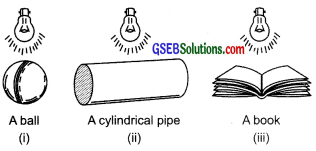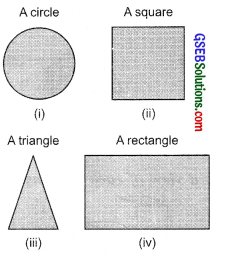# GSEB Solutions Class 7 Maths Chapter 15 Visualising Solid Shapes Ex 15.4

Gujarat Board GSEB Textbook Solutions Class 7 Maths Chapter 15 Visualising Solid Shapes Ex 15.4 Textbook Questions and Answers.

## Gujarat Board Textbook Solutions Class 7 Maths Chapter 15 Visualising Solid Shapes Ex 15.4Question 1.
A bulb is kept burning just right above the following solids. Name the shape of the shadows obtained in each case. Attempt to give a rough sketch of the shadow. (You may try to experiment first and then answer these questions).Solution:
When light falls just above the solid, i.e.
(i) A ball, the shadow looks like a circle.
(ii) A cylindrical pipe, the shadow looks like a rectangle.
(iii) A book, the shadow looks like nearly a rectangle.Question 2.
Here are the shadows of some 3 -D objects, when seen under the lamp of an overhead projector. Identify the solid(s) that match each shadow. (There may be multiple answers for these!)Solution:
(i) The given shadow corresponds to a sphere.
(ii) The given shadow corresponds to a cube.
(iii) The given shadow corresponds to a cone.
(iv) The given shadow corresponds to a cuboid or a cylinder.Question 3.
Examine if the following are true statements:
(i) The cube can cast a shadow in the shape of a rectangle.
(ii) The cube can cast a shadow in the shape of a hexagon.
Solution:
(i) The given statement is true.
(ii) The given statement is false.